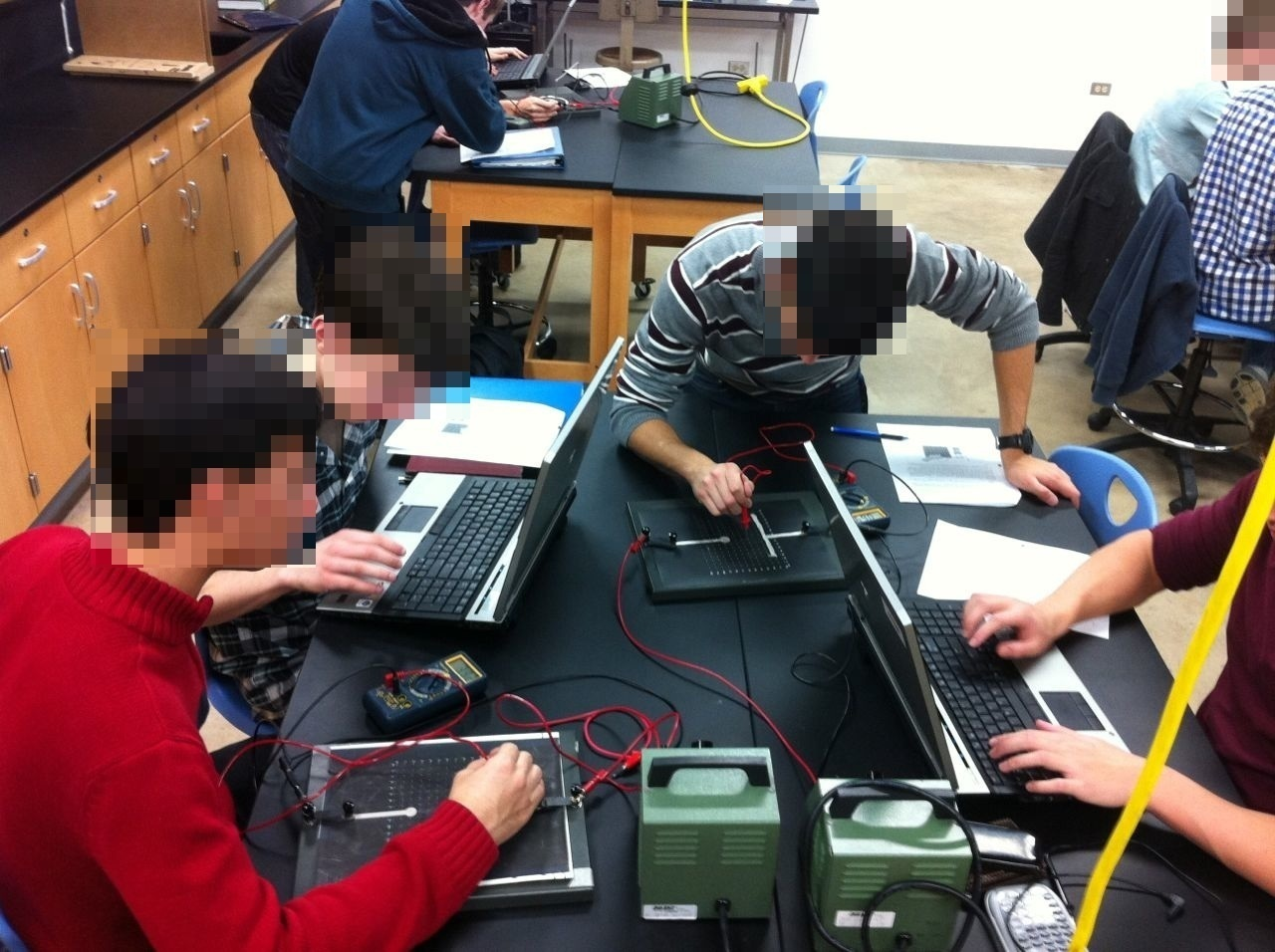# Day 95: Mapping Electric Potential

In AP Physics B, we spend a lot of time learning to visualize electric fields and equipotentials. One of the most helpful activities to develop this visualization is a lab where students map the electric potential on a grid of conductive paper and enter the results in an Excel spreadsheet that generates a 2D contour plot and a 3D surface plot. (This is the one time during the year we use Excel.) The 3D surface plot is particularly good at helping students visualize the electric field and equipotentials. We use a modified version of the spreadsheet that comes with the Advanced Physics with Vernier – Beyond Mechanics Lab BookI believe this surface plot is of two concentric circles (high potential is the inner circle).